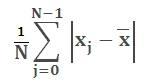# SQL Server average deviation function

Updated: 6 August 2010

Use AVEDEV_q to calculate the average of the absolute deviations of data points from their mean. The equation for average deviation isSyntax
SELECT [wctStatistics].[wct].[AVEDEV_q] (
<@Known_x_RangeQuery, nvarchar(4000),>)
Arguments
@Known_x_RangeQuery
the select statement, as text, used to determine the known x-values to be used in the AVEDEV_q calculation.
Return Types
float
Remarks
·         No GROUP BY is required for this function even though it produces aggregated results
·         For simpler queries, consider using the AVEDEV function
Examples

To determine the average deviation for all students in all subjects:

This produces the following result

----------------------
3.55294214876033

(1 row(s) affected)

To calculate the average deviation for each subject

SELECT Distinct s.subject, wct.AVEDEV_q('Select grade from #s1 where #s1.subject = ' + char(39) + s.subject + char(39))
from #s1 s

This produces the following result

subject
-------------------------------------------------- ----------------------
Foreign Language                                   4.04181818181818
History                                            2.215
Literature                                         2.215
Math                                               3.25818181818182
Science                                            2.73727272727273

(5 row(s) affected)

To calculate the average deviation for each student

SELECT distinct s.student
,wct.AVEDEV_q('Select grade from #s1 where student = ' + char(39) + s.student + char(39))
from #s1 s

This produces the following result

student
-------------------------------------------------- ----------------------
Student 01                                         0.520800000000003
Student 02                                         1.1032
Student 03                                         1.34
Student 04                                         1.5128
Student 05                                         1.6472
Student 06                                         1.7656
Student 07                                         1.8744
Student 08                                         1.9752
Student 09                                         2.0672
Student 10                                         2.1576
Student 11                                         2.248
Student 12                                         2.336
Student 13                                         2.4232
Student 14                                         2.5128
Student 15                                         2.604
Student 16                                         2.7016
Student 17                                         2.79919999999999
Student 18                                         2.9128
Student 19                                         3.0344
Student 20                                         3.1808
Student 21                                         3.3696
Student 22                                         3.6528

(22 row(s) affected)

### SupportCopyright 2008-2021 Westclintech LLC         Privacy Policy        Terms of Service